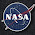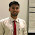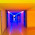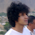## November 9, 2015

### Explaining the Hybrid A Star pathfinding algorithm for selfdriving cars

Let's say you are standing somewhere in a room and would like to find the shortest path to a goal. You can see a few obstacles, such as a table, that you would like to avoid. The easiest way to solve the problem (if you are a computer) is to divide the room into many small squares (cells) and then use the common A* (A Star) search algorithm to find the shortest path. But what if you are a car and can't turn around 360 degrees like a human can, then you have a problem! Well, at least until you learn the Hybrid A Star search algorithm. With that algorithm you will be able to find a drivable path to the goal.

The reason I wanted to learn the Hybrid A* algorithm was that I took a class in self-driving cars, where the teacher showed a really cool video with a car that drove around in a maze until it found the goal:

The problem was that the teacher didn't explain the Hybrid A* algorithm - he just explained the classic A* algorithm, so I had to figure it out on my own. This took some months, so I decided to write a summary of what I learned.

Before you begin, you have to learn the "normal" A* algorithm. If you don't know where to begin, I suggest you take the same class I took: Artificial Intelligence for Robotics - Programming a Robotic Car. It's free to take it, so don't worry. You should also read the sources I used to figure out the algorithm, mainly the following reports:

While those reports above will explain the more complicated algorithm that will be a little faster and maybe produce a better result, I will explain the very basic idea so you can get started. And when you can understand the basic idea behind the Hybrid A* search algorithm you can improve it.

### Hybrid A Star

First of all you need to learn how to simulate a vehicle that you can drive with the help of math. There are several car models, but you can learn the ideas behind the one I used by watching this video from the class I took (I had to replace the sin with cos and vice versa when I converted the math to from Python to Unity because of Unity's coordinate system, so you might have to experiment a little):

If you want to attach a trailer to the car, there's a mathematical model you can use for that as well: A car pulling trailers. And if you investigate the area further, you will also find models for odd cars that steer with both the front and rear wheels, so you are never limited by the simulation models. The tricky part is to try to replicate these simple models by using a real car when you have found a path, but that's a later problem!

Next step is to watch this video where the teacher Sebastian Thrun is explaining the basic idea behind the Hybrid A Star algorithm. The video is actually from the basic course in Artifical Intelligence, which is funny because he didn't explain it in the more advanced course.

The problem with the video is that Sebastian Thrun is just drawing one line from the first square, even though he should have drawn several lines (one for each turning angle). According to the reports from above, you should simulate three angles: maximum steer left, maximum steer right, and forward. I'm currently using the following steering angles: [-40, 0, 40]. The driving distance (d) is the same as the hypotenuse of one square plus some small distance so you never end up in the same cell again.

So from each node, you drive forward with a distance of d with three different angles, and if the car can reverse, you need to reverse with the same distance and three angles. So from each node, you end up with 6 child nodes. For each child, you have to calculate the cost (g-cost) and heuristic (h-cost). The self-driving car Junior used a more complicated heuristic, but you can use the traditional Euclidean distance as the heuristic before you begin calculating something more advanced.

You also need a few extra costs. According to the reports, you should add an extra cost to those nodes that change steering angle compared with the previous node, so you have to save the steering angle in each node. You should also add an extra cost if the node is close to an obstacle, or if you are reversing. The result is that you prioritize nodes that avoid obstacles because obstacles are bad and may scratch the car's paint.

Now you have 6 child nodes and have to investigate if they are valid. A node is of course not valid if it's colliding with an obstacle or is outside of the map, so you have to check that. Neither is a node valid if you have closed the cell it's in (after previously removing a node from the list of open nodes). The way you close nodes is that for each cell you close it if the car has not been there before with a certain heading angle. So you need to determine a heading resolution for each cell (I believe the report is using 5 degrees but I'm using 15 degrees or you will end up with a lot of nodes). Each child node has a heading, so you need to round that heading to the heading resolution you determined. Then you need a data structure so you can close a cell with a specific heading. So the car can move in to a cell if it has not been there before with that rounded heading.

All valid child nodes should now be added to the list of open nodes (like in normal A star). The biggest problem I had from a performance point of view was the search among the open nodes for the node with lowest f-cost. After a few attempts with different algorithms I realized that a heap was the fastest algorithm.

When you remove a node from the list of open nodes, you need to check if it has found the path to the goal. It will never find the exact path, so you need to check if it's close to the goal by comparing the node's position and heading with the position and heading you want.

That's it! If you add the ideas from above you will be able to make something that looks like this:

### Make it better

If you reed the original reports, you notice that they are using some methods to make the Hybrid A star faster. These methods include:

Reeds-Shepp paths
If the map you have had no obstacles, you wouldn't need Hybrid A* because you could use a Reeds-Shepp path, which is the shortest distance between two cars if the cars can reverse. I've written a separate article about them and they were so annoying to make so I've decided to give away the code for free: Download Reeds-Shepp paths for Unity in C#. You can use them in the Hybrid A* first as a heuristic instead of just Euclidean distance, and you can also use them when expanding nodes. So instead of 6 child nodes, you get 6 + 1 node from the shortest Reeds-Shepp path.

Flowfield
A flowfield is very similar to the traditional A* algorithm, but instead of finding the shortest path from one cell to another cell, the flowfield algorithm is finding the shortest path from all cells to one or more other cells. This is for example useful as heuristic in Hybrid A* because the flowfield is avoiding obstacles. So as heuristic you should use the maximum of Euclidean distance, Reeds-Shepp path, and the flowfield. It looks like this (red is far away and green is close):

Voronoi field
A voronoi field tells the distance to the closest voronoi edge and the closest obstacle. To create a voronoi field, you first need to create a voronoi diagram which tells the distance to closest obstacle (the border is also an obstacle). You can create it with the help of a flowfield algorithm:

Then you need to identify the voronoi edges which is the edges where the color meet in the image above. Then you use the flowfield algorithm a second time to find the distance from the voronoi edges to the obstacles, and when you have these distances you can use the formula from the reports to calculate the voronoi field:

If you have the voronoi field you can speed up collision detection because you now know for each cell the distance to the closest obstacle. You can also use it when calculating the costs for each node. You should add a cost if a node is close to an obstacle, but you also want to find the shortest path and don't want the car to drive a long distance around obstacles. The voronoi field will help you add a small cost if you are close to an obstacle but still far way so the car will not be "scared" of obstacles.

### Make the car follow the path

The path you get from the Hybrid A star is ugly. To make it easier for the car to follow it you need to smooth it by using some technique like gradient descent (which you will learn in the online class I recommended you should take). You might also need to add more waypoints between the original waypoints to make it easier for the car to follow it.

To make the car follow the path, you can use a PID controller. The problem is that you used the rear wheels of the car when making the path in the Hybrid A* algorithm. But the car wants a path following the front wheels to follow it easier. So you need to move the path forward a distance which is the distance between the front and rear axle (the wheel base).

To make the car reverse along the path you need to move the path in the opposite direction, so the reverse path can be said to be the "mirrored front path". When the car is reversing along the mirrored path, you use the mirrored wheel-base distance to calculate the error to the path. In the video below, this mirrored path is the green line, the red line is the original path, and the blue line is the path the vehicles are using when driving forward.

And if everything works fine, it should look like this:

I believe Tesla is using something similar in their Summon feature algorithm, because they look very similar from above. But in the video you can see that the Tesla is is also following traffic rules (driving in the right the lane, etc). To add that you can maybe add a weight so if the vehicle is driving with the wrong direction in a lane then the node gets a lower priority, but that's for another update!

1.Great work with Unity. I was looking for this explanation. Thanks.

2.Why don't you please post the source code for the hydrid A* algorithm, like you do for your other tutorials.

1.Because there's only 24 hours a day and the code is a bit messy, but you can send me an email and I will reply with the project

3.Can you please comment on the grid size/ work space dimensions you are considering ?

4.Can you explain how to use PID controller to follow that path?
Thanks alot

5.First of all thanks for your tutorials/ I find very usefull boat tutorial/ but find that the form of collider play big role in simulation of boat. And waves generated with collider don't
interacted with underwater mesh.
If its not difficult for please sent source of pathfind in Unity.
geotyper@gmail.com
Thanks
Alexander

1.sorry waves generated with shader not collider

6.Hi,
I am also implementing this algorithm. In case you want to check out: https://github.com/trgtylcnky/global_planner_turgut

The problem I am facing of is getting to the final. In your simulation, I see a path consists of two arc and one line is calculated. I cannot calculate this unfortunately (I am surprised of my lack of geometry skills). Can you give me a formula or any other help of calculating that?

Thank you.

1.The path is called a Reed-Shepp path. It's a generalization of the Dubins path. Implementing it is not hard but it would probably take a lot of time. It's easier/faster if you go with Dubins path.

7.did the source code written by c++

1.Noooooooo, it's written by me in c# :P

2.how do you set up vironoi field with help of flowfield

3.You start from each obstacle cell and then you flow out while checking if the cell you are flowing to is not closer to another obstacle!

4.yep,but how do i find vironoi edge? Check cell whose next cell's nearest obstacle cell is not same?

5.6.8.9.